# Excel formulas not working, not updating, not calculating: fixes & solutions

This tutorial explains the most common mistakes when making formulas in Excel, and how to fix a formula that is not calculating or not updating automatically.

Can someone imagine using Microsoft Excel without formulas? I believe no one can. And hardly anything could compare to the frustration caused by Excel formulas stop working all of a sudden. When this happens, a bunch of questions immediately flash across your mind. Why is my Excel formula not calculating? Why doesn't this formula update its value automatically? Is my Excel corrupt or is this due to some malicious virus? And how do I get my Excel to calculate and update formulas automatically again? Don't worry, most likely your Excel is all right, and you will get all the answers in a moment.

## Excel formulas not working

Symptoms: Excel formula not working correctly, it returns an error or a wrong result.

This section provides a summary of the most common mistakes people make when creating formulas in Excel and solutions to fix them.

#### 1. Match all opening and closing parentheses in a formula

As you know, the arguments of Excel functions are entered within the parentheses. In complex formulas, you may need to enter more than one set of parentheses, one within another, to indicate the order in which the calculations should take place. When creating such a formula, be sure to pair the parentheses properly so that you always have a right parenthesis for every left parenthesis in your formula.

Microsoft Excel displays the parentheses pairs in different colors as you enter them in a formula. If your formula is short of one or more parentheses, Excel displays an error message and suggests a correction to balance the pairs. Please see How to highlight and match parenthesis pairs for more information.

#### 2. Enter all required arguments in an Excel function

All Excel functions have one or more required arguments. Some functions also have optional arguments, which are enclosed in [square brackets] in the formula's syntax.

A formula must contain all of the required arguments, otherwise Excel displays "You've entered too few arguments for this function" alert.

If you have entered more arguments than allowed by the formula's syntax, you will get "You've entered too many arguments for this function" error message.

#### 3. Do not nest more than 64 functions in a formula

When nesting two or more Excel functions into each other, e.g. creating a nested IF formula, remember about the following limitations:

• In Excel 2016, Excel 2013, Excel 2010 and Excel 2007, you can use up to 64 nested functions.
• In Excel 2003 and lower, only up to 7 nested functions can be used.

#### 4. Don't enclose numbers in double quotes

In Excel formulas, any value enclosed in double quotes is interpreted as a text string.

What it means is that if you enter a formula like `=IF(A1>0, "1")`, Excel will treat number 1 as text, and therefore you won't be able to use the returned 1's in other calculations. To fix this, just remove the double quotes around "1": `=IF(A1>0, 1)`.

So, whenever you are writing a formula for numerical values, follow this simple rule: don't enclose numbers in double quotes unless you want them to be treated as text.

#### 5. Enter numbers without any formatting

When using a number in an Excel formula, don't add any decimal separator or currency sign like \$ or €.

Remember that in Excel formulas, a comma is typically used to separate a function's arguments, and the dollar sign makes an absolute cell reference.

For instance, instead of entering \$50,000 in your formula, input simply 50000, and use the Format Cells dialog (Ctrl + 1) to format the output to your liking.

#### 6. Make sure numbers are not formatted as text values

Numbers formatted as text values are another common reason for Excel formulas not working. At first sight, they look like normal numbers, but Microsoft Excel perceives them as text strings and leaves out of calculations.

The visual indicators of text-numbers are as follows:

• Numbers formatted as text are left-aligned by default, while normal numbers are right-aligned in cells.
• The Number Format box on the Home tab in the Number group displays the Text format.
• When several cells with text numbers are selected on the sheet, the Status Bar only shows Count, while usually it shows Average, Count and SUM for numbers.
• There may be a leading apostrophe visible in the formula bar, or green triangles appear in the top-left corner of the cells.

The below screenshot shows that even a simple Excel SUM formula may not work because of numbers formatted as text: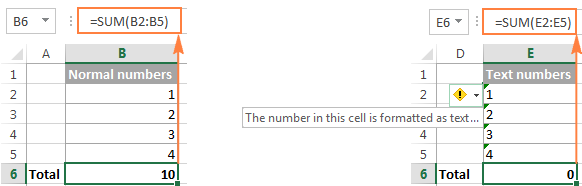To fix this, select all problematic cells, click the warning sign, and then click Convert to Number: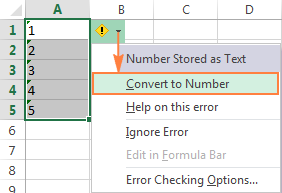In some cases, however, neither green triangles nor the warning sign appear in cells. For example, if you enclose numeric values in double quotes in your formulas, Excel assumes you want to output a text string rather than a number.

At first sight, the following formula appears to be working fine:
`=IF(A1="Good", "1", "0")`

But the problem is the returned 1's and 0's are text values, not numbers! And if you reference any cells with the above formula in other formulas, those cells won't be included in calculations. As soon as you remove "" surrounding 1 and 0 in the above formula, Excel will treat the outputs as numbers and they will be calculated correctly.

If the small green triangles do not appear in cells for some other reason, look at the Number Format box on the Home tab in the Number group. If it displays Text, try clearing all formatting for the problematic cells, and set the cells' format to Number or General. If that doesn't work, you might have to create a new column, manually input the data (e.g. copy your text-numbers to Notepad, and then back to a new column), and delete the broken column.

Another possible solution is to multiply the values in the problematic column by 1 using a simple formula like `=A1*1`. And then, copy the formula cells and paste them as values in the same or in any other column via Paste Special > Values.

#### 7. Separate function arguments with a proper character

Most of us are used to separating function arguments with commas. However, this does not work for everyone's Excel. The character you use to separate arguments depends on the List Separator set in your Regional Settings.

Comma is the default list separator in North America and some other countries. In European countries, comma is used as the decimal symbol and the list separator is usually set to semicolon.

For example, in North America you would write `=IF(A1>0, "OK", "Not OK")`, while European users of Excel should put the same formula as `=IF(A1>0; "OK"; "Not OK")`.

So, if your Excel formulas are not working because of "We found a problem with this formula..." error, go to your Regional Settings (Control Panel > Region and Language > Additional Settings) and check what character is set as List Separator there. And then, use exactly that character to separate arguments in your Excel formulas.

#### 8. Enclose workbook and worksheet names in single quotes

When referring to other worksheets or workbooks that have spaces or non-alphabetical characters in their names, enclose the names in 'single quotation marks'. For example,

Reference to another sheet:
`=SUM('Jan Sales'!B2:B10)`

Reference to another workbook:
`=SUM('[2015 Sales.xlsx]Jan sales'!B2:B10)`

#### 9. Include the full path to a closed workbook

If you are writing a formula that references a closed Excel workbook, your external reference must include the workbook name and entire path to the workbook. For example:

`=SUM('D:\Reports\[Sales.xlsx]Jan'!B2:B10)`

If the above tips do not help, try to evaluate and debug each part of your formula individually by using the F9 key and other debugging techniques explained in the following tutorial: How to evaluate and debug formulas in Excel.

## Excel formulas not updating

Symptoms: The value returned by your Excel formula does not update automatically, i.e. the formula continues to show the old value even after you've changed the values of the dependent cells.

When Excel formulas are not updating automatically, most likely it's because the Calculation setting has been changed to Manual instead of Automatic. To fix this, just set the Calculation option to Automatic again.

On the Excel ribbon, go to the Formulas tab > Calculation group, click the Calculation Options button, and select Automatic: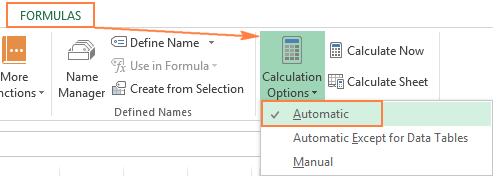Alternatively, you can change this setting in Excel Options:

• In Excel 2003, click Tools > Options > Calculation > Calculation > Automatic.
• In Excel 2007, click Office button > Excel options > Formulas > Workbook Calculation > Automatic.
• In Excel 2010, Excel 2013, and Excel 2016, go to File > Options > Formulas > Calculation options section, and select Automatic under Workbook Calculation.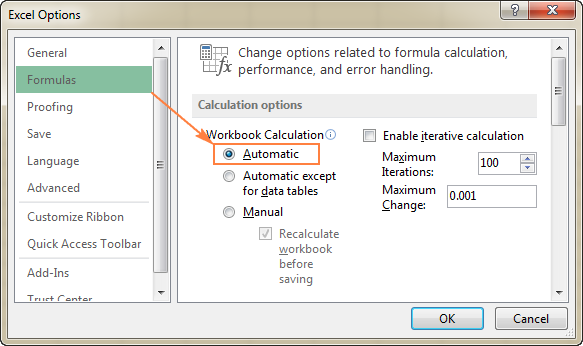### How to force Excel formulas to recalculate

If for some reason, you need to have the Calculation option set to Manual, you can force the formulas to recalculate by clicking the Calculate button on the ribbon or by using one of the following shortcuts:

To recalculate the entire workbook:

• Press F9, or
• Click the Calculate Now button on the Formulas tab > Calculation group.

To recalculate an active sheet:

• Press Shift + F9, or
• Click Calculate Sheet on the Formulas tab > Calculation group.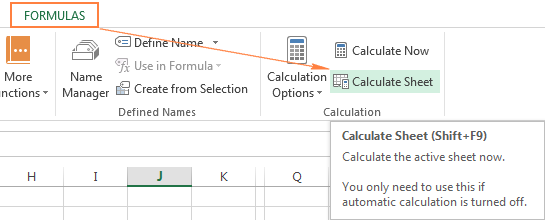To recalculate all sheets in all open workbooks, press Ctrl + Alt + F9.

If you need to recalculate only one formula on a sheet, select the formula cell, enter the editing mode either by pressing F2 or double clicking the cell, and then press the Enter key.

## Excel formulas not calculating

Symptoms: A cell displays the formula, not the result.

If your Excel formula is not working because a cell displays the function instead of the calculated value, it's because one of the following reasons.

#### 1. Show Formulas mode is turned on

The most common reason for an Excel formula not calculating is that you have inadvertently activated the Show Formulas mode in a worksheet.

To get the formula to display the calculated result, just turn off the Show Formulas mode by doing one of the following:

• Pressing the Ctrl + ` shortcut, or
• Clicking the Show Formulas button on the Formulas tab > Formula Auditing group.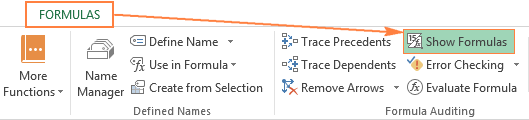#### 2. A formula is entered as text

Another frequent reason for your Excel formula not calculating is that the formula has been formatted as text. To check this, select the formula cell, and look at the Number Format box in the Number group on the Home tab: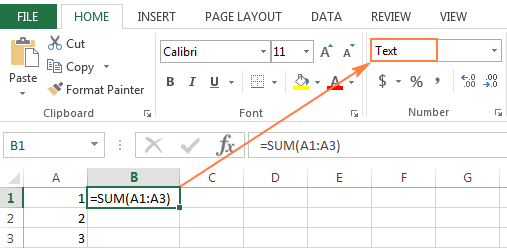If it is the case, change the cell format to General, and while in the cell press F2 and Enter for the formula to recalculate and display the calculated value.

#### 3. A formula cell has a leading space or apostrophe before the equal sign

If you have inadvertently entered a space or apostrophe (') before the equal sign, Excel treats the cell contents as text, and consequently does not evaluate any formula within that cell (a leading space often appears when you copy a formula from the web). To fix this, just remove the leading space or single quote.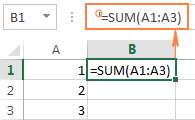This is how you deal with formulas not working in Excel. If you know any other solutions to fix formulas not updating or not calculating, please do share in comments. I thank you for reading and hope to see you on our blog next week.

### 275 responses to "Excel formulas not working, not updating, not calculating: fixes & solutions"

1.Aftab Alam Khan says:

Thanks a lot. It is Really very helpful. My issue was resolved within some seconds.

2.barb says:

I am trying to use IF function to determine if numbers in two cells are the same. I think the issue is one of the cells in the comparison is from a calculation. i.e. Cell A (\$0.14) is a hard number typed in, Cell D is calculated from numbers in cell B and Cell C (B-\$31.21*0.45%).
The IF function will only work if I switch the "if true" and "if false" - that is completely backwards and doesn't make sense to do. Any guidance is appreciated.

•Alexander Trifuntov (Ablebits.com Team) says:

Hello!
I recommend using rounding to 2 decimal places in a cell with a formula. Read about rounding functions here.

•Missen says:

You just saved my sanity with that answer :-)

3.Hylke says:

I'm trying to find the reason that certain cells do not generate an outcome when my colleague opens the sheet, whereas with me and other colleagues it does. His setting is set at automatic, so that's not it. We've also restarted excel. It's a string of cells that return a value by looking it up in a database. (e.g. array functions and vlookup involved)
Does anyone have any suggestions where to look?

•Alexander Trifuntov (Ablebits.com Team) says:

Hello!
You haven't written what kind of data you're working with. Perhaps you have differences in the regional settings, in the separators used. If you give more information, I will try to help.

4.Jim says:

In my spreadsheet, there are a couple of random cells where the formula does not produce the correct result. It's a simple if statement.

•Alexander Trifuntov (Ablebits.com Team) says:

Hello!
Please specify what you were trying to find, what formula you used and what problem or error occurred.

5.reza says:

Thanks a lot

6.vahid says:

thank you it helps.

7.BISHNU SUBEDI says:

ITS REALLY HELPFULL THANKS FOR THIS.

8.Maricela says:

Found the solution to my problems. Thanks

9.Brandon says:

Hi, I'm trying to produce a Gantt chart and have been following some online tutorials (Vertex42)

Having carefully followed the instructions it appears l am unable to fill the bars - all relevant information is inputted - weekly dates, start dates, and finished dates as like the tutorial.
However when l select conditional formatting, new rule and input the new formula - AND(E\$5>=\$B7,E\$5<=\$C7), followed by pressing format which then gives the option to select fill colour, on selection of fill colour it returns to format options - where l select "OK" and then nothing changes, unlike the tutorial where all relevant information bars highlighted in the chosen colour

10.Mike says:

some fields recalculate when I change a variable, some don't. When I try to fix a field individually by re-inputting the formula the value goes to 0.

I looked into the fixes listed here with no luck.

11.N says:

I am trying to add a simple column of numbers and sum it up in a separate cell - like I've done for 20 years. I am typing : =SUM(highlighting the cells).... It simply does not add up the result, just showing 0! Ditto any other function like AVERAGE.I followed your tips to no avail. I am using licensed 365 Excel subs. (Also how may I simply clear all formatting from all cells? My cells are all General.

12.adrian says:

was using excell all was ok now suddenly any new formula eg sum of 2 cells or sum of row, and all gives a \$ - result. how can i fix this?

13.Marco says:

Hi,
I have an own function, eg :

Function Pipo( nRow as integer, nColumn as Integer, nVal as Integer )
Cells( nRow, nColumn+1).Value = nVal+1
Pipo = nVal
End Function

-> Update next Cell with value : nVal+1
-> Returns : nVal

The cell it is used in in looks like : =Pipo( ROW(), COLUMN(), 9 )

However the return in "#VALUE" and the next cell is not updated.
It is the Cells function that is causing this error. Even using Range() instead does not work.

Any ideas?

G,
Marco

•Marco says:

Well, found it -> updating a cell only works from within a Subroutine.

Case closed.

14.Eunseo says:

Thanks so much!! I was on the verge of giving up and found an easy solution here.

15.Aga says:

Hallo,
Could you help me with this formula, please?
=IF(AND(F14=12),"Continue",IF(AND(F147),"Continue",IF(AND(F14>70,F22>=12),"Rethink","Cancel")))
In place where supposed to appear "Rethink" stubbornly apeears "Continue". I tried many different options but even when I delete everything and left only two parts and "Cancel" as false statement , it showed me "Continue"
There are 5 columns for this formula. Four are working good. All changes in figures cause changes in results - the text. The last column resists.This part : IF(AND(F14>70,F22>=12),"Rethink" is designed exactly for this column to met all condition. I tried everything I know and it is not working. Could you help me with this - why it is happening? I checked settings in my excel and everything is ok

•Alexander Trifuntov (Ablebits.com Team) says:

Hello!
Your formula is incomplete. Try to insert spaces before and after the> and <. Then it will be recorded in full.

•Aga says:

Hallo, Thank you for response, Btw I just noticed I made the mistake when I was copying my formula to show you, here is right one =IF(AND(F1412),"Continue",IF(AND(F147),"Continue",IF(AND(F14>70,F22>12),"Rethink","Cancel"))), I added spaces as you advised and got info that typo was found and excel corrected it back with no space :( still not working

•Alexander Trifuntov (Ablebits.com Team) says:

Hello!
Unfortunately, I cannot figure out your formula. I forgot to say that spaces should not be inserted in Excel, but in the comment itself. If possible, describe in words the conditions in the formula to make it easier for me to understand how it works. Thank you.

16.Trevor l says:

Would a formula not work due to an enabled editing from a protected document? the person who sent me the excel document via email can edit the worksheet and use the formula function after enable editing. although when i open the same document from the email, I do not get the "enable editing" yellow bar and the document's formulas do not work. Please help?

17.Abby says:

Formula for a cell is not calculating, it just shows as zero. I'm using accounting format, but I have attempted to switch to general and it still doesn't calculate. It is an extremely basic formula - =B14+F14. I sent the email to a coworker and she is having the same issue.

18.Donbocso says:

Hi, my problem is I just Sumif formula for using one file to another file, if both files are open that formula give a correct ans. but i closed that Conciliated file that formula shows #value!. Please advise.

19.Donna Hooper says:

I'm having the same issue as Abby - I can't get a simple AutoSum formula to work - it just shows a zero. I've tried both ways (=B21+B40) and =(SUM(B1, B40). And when I click on prior AutoSum formulas that worked perfectly, they reset to zero.

20.Michael says:

I am using MS Office and Excel for Mac; do the above fixes differ when trying to get Excel to calculate formulas? I came here looking for an answer to the issue of the fact that I just noticed that my Excel spreadsheet in not automatically updating and maintaining old data in cells after I have changed the data.

My only option right now is to re-calculate every cell and every calculation. but then when I make a change I'll have to watch changes are made again.

The fix recommendation of making sure my Formulas, Options, setting is set for "Calculate Automatically" has been tried...no different result.

Does anyone have any other ideas on what is wrong and a fix?

21.Cuma says:

Thank you for your suggestion to check the List separator (Suggestion#7).
I have been using vlook every day for nearly 2 years but recently my excell was updated and my Vlookup wasn't working and I was going crazy and finally realized the list separator is not comma but | in the new version
Thank you.

22.Andras says:

Hi! I have problem with the auto calculation issue. I use an MS Form what send data to a OneDrive shared excel file. This is works fine. I use some more analysis and calculation from the received data. And here is the problem , since the data is not calculated even with F9 recalculate function. However if I'm open the cell function and enter on, than the calculation is working. It is not the way to make manual open-enter on all cell, so I really looking after where is the bug.
Really I don't understand the issue with this shared xls and struggling with solution!
Many thanks for suggestions!

23.Tina says:

Hi, I'm trying to collate attendance to meetings that are being run each day. My colleagues are generating a 'Register' spreadsheet from a meeting attendance download in TEAMS. It just gives three columns of Name, Action, Date/time stamp. This is changed from a CSV to Excel Workbook.
My 'Attendance' Excel work book has the following formulae:
=IF(IFERROR((COUNTIFS('filestructure\[2021-01-11 P2 Po Hs.xlsx]meetingAttendanceList (2)'!\$A:\$A,A105,'filestructure\[2021-01-11 P2 Po Hs.xlsx]meetingAttendanceList (2)'!\$B:\$B,"Joined")),0)>=1,1,0)
If I open the 'Register' my 'Attendance' calculations work but, if the 'Regsiter' is closed I just get 0 for attendance when it should be 1
I have to link into a lot of different 'Registers' for each day and am currently opening all the registers in order to get some figures into my 'Attendance' spreadsheet. What have I done wrong???
I've got the 'Attendance' and 'Register' spreadsheets in the same folder on our network. It's a lengthy location so I've reduced it to "filestructure" for your sanity. The fact that it calculates it when I open the register tells me it can find it. Very confused - HELP!!!

•Alexander Trifuntov (Ablebits.com Team) says:

Hello!
If I understand your task correctly, your formula uses data from a separate file. She can get this data only when this file is open in Excel. If the file is not open in Excel, then the data from it cannot be used in formulas.

•Tina says:

That's right.

24.Stefanita Toader says:

Hello, i have an excel document and in some cells, like this: =FRONTPAGE!B15. From the beginning all was ok, instead formula, the corresponding text from sheet FRONTPAGE, cell B5 was displayed. But now if I double click on that cell containing formula, the corresponding text is replaced by formula.
Calculation option is set to Automatic.

25.Daniell T says:

I have simple sum formulas on 3 spreadsheets. I created a 4th document (recon) to compare the totals pages to the prior 3 spreadsheets. If I open the recon spreadsheet in one window, and the other 3 spreadsheets in another window, the formulas don't automatically update. They will only update if I have all spreadsheets open in the same window. Is there a workaround or is this just how it needs to be?

•Alexander Trifuntov (Ablebits.com Team) says:

Hello!
Unfortunately I was unable to reproduce your problem. A separate window is always opened for each file. If a file is opened in Excel, then the data from it is always updated in another file.